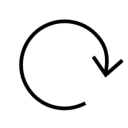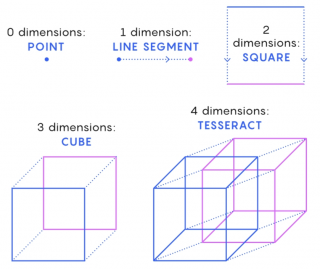dimension ⪢⪢
The number of _____s is how many values are needed to locate points on a shape. #math
http://qindex.info/i.php?x=10324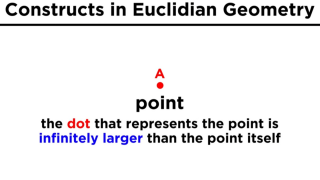○○○ Point ⪢⪢
First we look at a point. This is nothing more than a location in space. It is zero-dimensional, meaning that it has no dimensions of any kind. #math
http://qindex.info/i.php?x=5030dimension
The number of dimensions is how many values are needed to locate points on a shape.
http://qindex.info/i.php?x=5044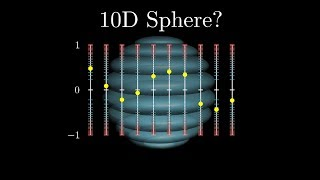Thinking visually about higher dimensions
http://qindex.info/i.php?x=3388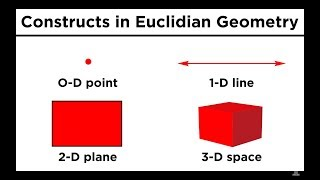◌◌◌ Line
From here, we can construct a one- dimensional object by stringing an infinite number of points along a particular dimension. This object is called a line.
http://qindex.info/i.php?x=5177◌◌◌ Space
By stringing an infinite number of lines along a dimension perpendicular to the line, a two-dimensional object called a plane can be obtained. And then if we string an infinite number of planes in either direction, we get three dimensional space.
http://qindex.info/i.php?x=5178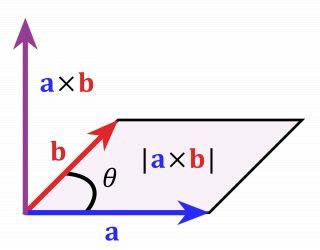Cross Product
Given two nonzero vectors in two or three dimensions, their cross product is a vector with magnitude equal to the product of the magnitudes of the vectors times the sine of the angle between the vectors and direction perpendicular to the vectors.
http://qindex.info/i.php?x=5291◌◌◌ Plane
By stringing an infinite number of lines along a dimension perpendicular to the line, a two-dimensional object called a plane can be obtained. And then if we string an infinite number of planes in either direction, we get three dimensional space.
http://qindex.info/i.php?x=5031Leonard Susskind
The space itself may be more than three dimensions. But we can't visualize more dimensions. The architecture of the brain itself is evolved in the world of three dimensions. We only describe more dimensions by pure mathematics.
http://qindex.info/i.php?x=5242dimension
The number of dimensions is how many values are needed to locate points on a shape.
http://qindex.info/i.php?x=9579
-----